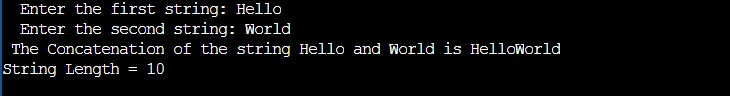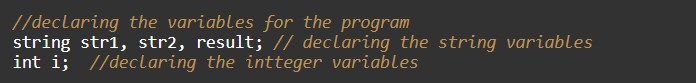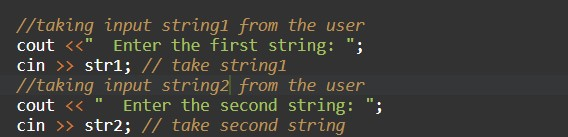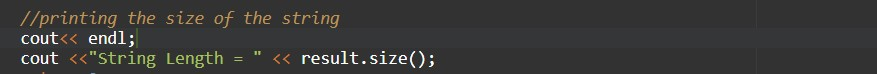# C++ Program to Find length of String

In this tutorial you will learn about the C++ Program to Find length of String and its application with practical example.

## C++ Program to Find Length of String

In this tutorial, we will learn to create a C++ program that will Find the length of String in C++ programming.

## Prerequisites

Before starting with this tutorial we assume that you are best aware of the following C++ programming topics:

• Operators in C++ Programming.
• Basic Input and Output function in C++ Programming.
• Basic C++ programming.
• Using Strings in C++ Programming.

## Program to Find the length of String

In this program, we will take the input as a string from the user and then we will find the length of the input string. Using the predefined string function from the c++ libraries.

With the help of this below program, we can find the length of the String.

## Output:-In the above program, we have first initialized the required variable.• str1 = it will hold the string value.
• str2 = it will hold the string value.
• result = it will hold the string value.
• i = it will hold the integer value.

Taking Input string from the user for copying the string.Printing the output size of the string.In this tutorial we have learn about the C++ Program to Find length of String and its application with practical example. I hope you will like this tutorial.